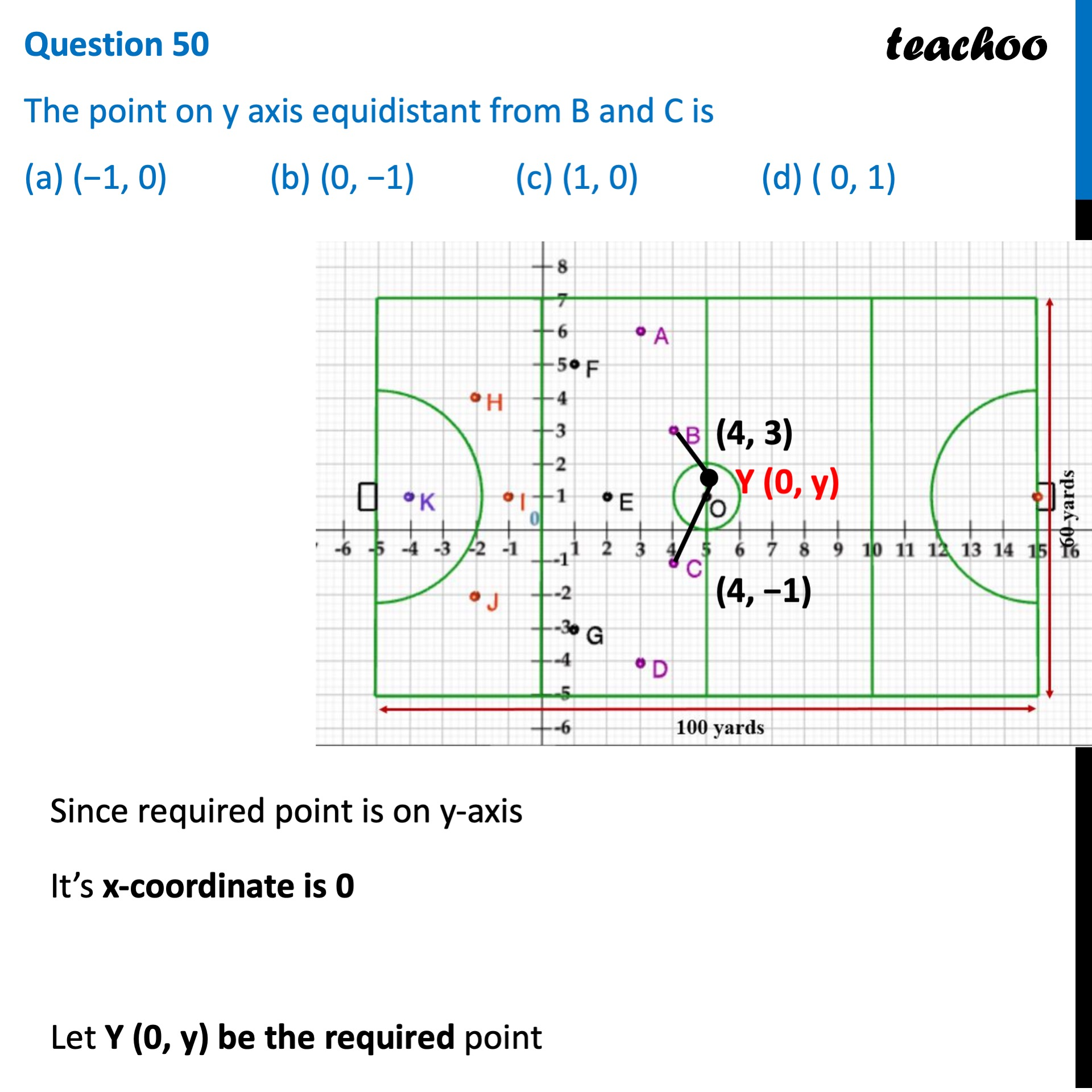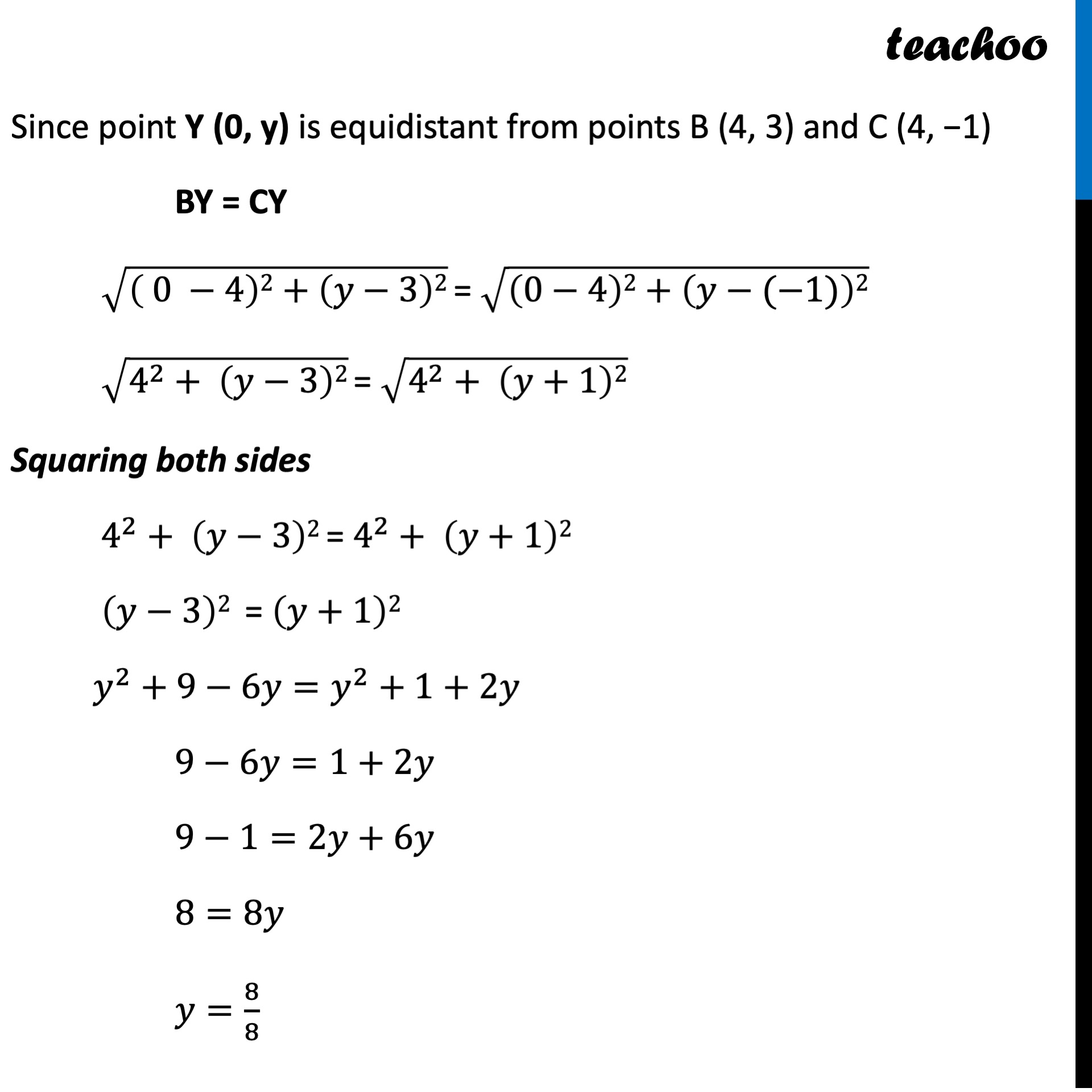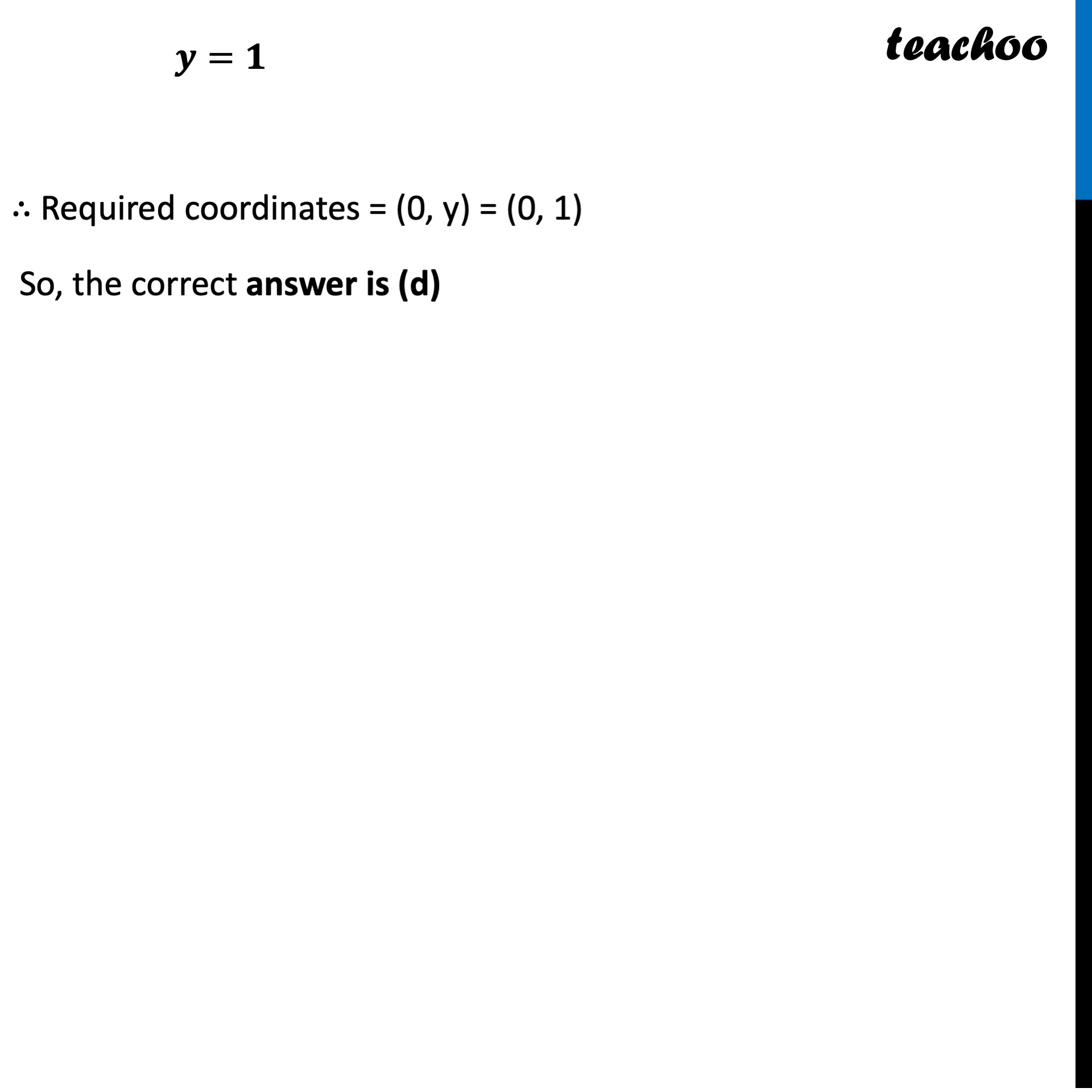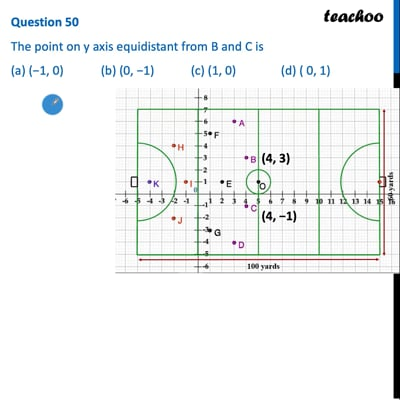CBSE Class 10 Sample Paper for 2022 Boards - Maths Standard [MCQ]

Class 10
Solutions of Sample Papers for Class 10 Boards

## (a) (−1, 0)   (b) (0, −1)   (c) (1, 0)   (d) ( 0, 1)This video is only available for Teachoo black users

Introducing your new favourite teacher - Teachoo Black, at only ₹83 per month

### Transcript

Question 50 The point on y axis equidistant from B and C is (a) (−1, 0) (b) (0, −1) (c) (1, 0) (d) ( 0, 1) Since required point is on y-axis It’s x-coordinate is 0 Let Y (0, y) be the required point Since point Y (0, y) is equidistant from points B (4, 3) and C (4, −1) BY = CY √(( 0 −4)2+(𝑦−3)2) = √((0−4)2+(𝑦−(−1))2) √(4^2+ (𝑦−3)2) = √(4^2+ (𝑦+1)2) Squaring both sides 4^2+ (𝑦−3)2 = 4^2+ (𝑦+1)2 (𝑦−3)2 = (𝑦+1)2 𝑦^2+9−6𝑦=𝑦^2+1+2𝑦 9−6𝑦=1+2𝑦 9−1=2𝑦+6𝑦 8=8𝑦 𝑦=8/8 𝒚=𝟏 ∴ Required coordinates = (0, y) = (0, 1) So, the correct answer is (d)